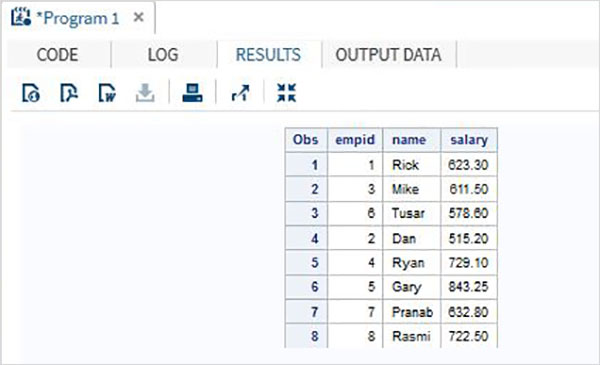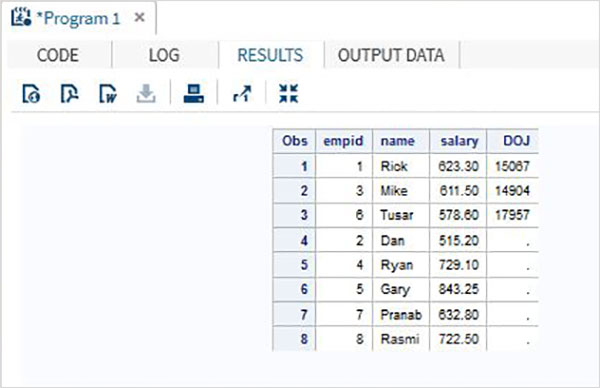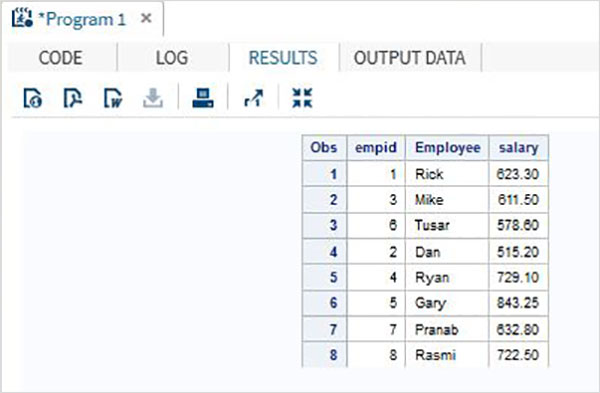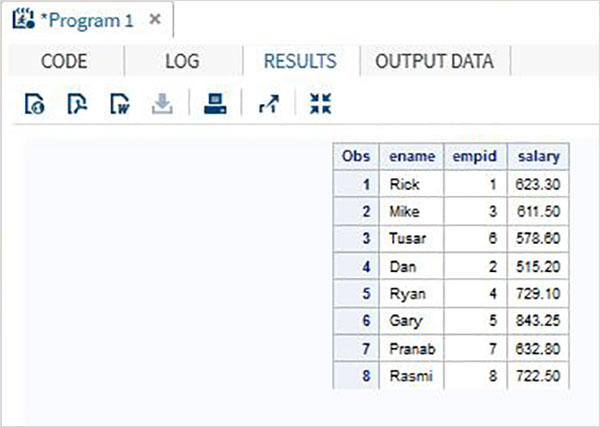# SAS - Concatenate Data Sets

Multiple SAS data sets can be concatenated to give a single data set using the SET statement. The total number of observations in the concatenated data set is the sum of the number of observations in the original data sets. The order of observations is sequential. All observations from the first data set are followed by all observations from the second data set, and so on.

Ideally all the combining data sets have same variables, but in case they have different number of variables, then in the result all the variables appear, with missing values for the smaller data set.

### Syntax

The basic syntax for SET statement in SAS is −

```SET data-set 1 data-set 2 data-set 3.....;
```

Following is the description of the parameters used −

• data-set1,data-set2 are dataset names written one after another.

### Example

Consider the employee data of an organization which is available in two different data sets, one for the IT department and another for Non-It department. To get the complete details of all the employees we concatenate both the data sets using the SET statement shown as below.

```DATA ITDEPT;
INPUT empid name \$ salary  ;
DATALINES;
1 Rick 623.3
3 Mike 611.5
6 Tusar 578.6
;
RUN;
DATA NON_ITDEPT;
INPUT empid name \$ salary  ;
DATALINES;
2 Dan 515.2
4 Ryan 729.1
5 Gary 843.25
7 Pranab 632.8
8 Rasmi 722.5
RUN;
DATA All_Dept;
SET ITDEPT NON_ITDEPT;
RUN;
PROC PRINT DATA = All_Dept;
RUN;
```

When the above code is executed, we get the following output.## Scenarios

When we have many variations in the data sets for concatenation, the result of variables can differ but the total number of observations in the concatenated data set is always the sum of the observations in each data set. We will consider below many scenarios on this variation.

## Different number of variables

If one of the original data set has more number of variables then another, then the data sets still get combined but in the smaller data set those variables appear as missing.

### Example

In below example the first data set has an extra variable named DOJ. In the result the value of DOJ for second data set will appear as missing.

```DATA ITDEPT;
INPUT empid name \$ salary DOJ date9.  ;
DATALINES;
1 Rick 623.3 02APR2001
3 Mike 611.5 21OCT2000
6 Tusar 578.6 01MAR2009
;
RUN;
DATA NON_ITDEPT;
INPUT empid name \$ salary  ;
DATALINES;
2 Dan 515.2
4 Ryan 729.1
5 Gary 843.25
7 Pranab 632.8
8 Rasmi 722.5
RUN;
DATA All_Dept;
SET ITDEPT NON_ITDEPT;
RUN;
PROC PRINT DATA = All_Dept;
RUN;
```

When the above code is executed, we get the following output.## Different variable name

In this scenario the data sets have same number of variables but a variable name differs between them. In that case a normal concatenation will produce all the variables in the result set and giving missing results for the two variables which differ. While we may not change the variable name in the original data sets we can apply the RENAME function in the concatenated data set we create. That will produce the same result as a normal concatenation but of course with one new variable name in place of two different variable names present in the original data set.

### Example

In the below example data set ITDEPT has the variable name ename whereas the data set NON_ITDEPT has the variable name empname. But both of these variables represent the same type(character). We apply the RENAME function in the SET statement as shown below.

```DATA ITDEPT;
INPUT empid ename \$ salary  ;
DATALINES;
1 Rick 623.3
3 Mike 611.5
6 Tusar 578.6
;
RUN;
DATA NON_ITDEPT;
INPUT empid empname \$ salary  ;
DATALINES;
2 Dan 515.2
4 Ryan 729.1
5 Gary 843.25
7 Pranab 632.8
8 Rasmi 722.5
RUN;
DATA All_Dept;
SET ITDEPT(RENAME =(ename = Employee) ) NON_ITDEPT(RENAME =(empname = Employee) );
RUN;
PROC PRINT DATA = All_Dept;
RUN;
```

When the above code is executed, we get the following output.## Different variable lengths

If the variable lengths in the two data sets is different than the concatenated data set will have values in which some data is truncated for the variable with smaller length. It happens if the first data set has a smaller length. To solve this we apply the higher length to both the data set as shown below.

### Example

In the below example the variable ename is of length 5 in the first data set and 7 in the second. When concatenating we apply the LENGTH statement in the concatenated data set to set the ename length to 7.

```DATA ITDEPT;
INPUT  empid 1-2 ename \$ 3-7 salary 8-14  ;
DATALINES;
1 Rick  623.3
3 Mike  611.5
6 Tusar 578.6
;
RUN;
DATA NON_ITDEPT;
INPUT  empid 1-2 ename \$ 3-9  salary 10-16 ;
DATALINES;
2 Dan    515.2
4 Ryan   729.1
5 Gary   843.25
7 Pranab 632.8
8 Rasmi  722.5
RUN;
DATA All_Dept;
LENGTH ename \$ 7   ;
SET ITDEPT  NON_ITDEPT ;
RUN;
PROC PRINT DATA = All_Dept;
RUN;
```

When the above code is executed, we get the following output.